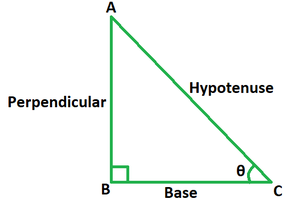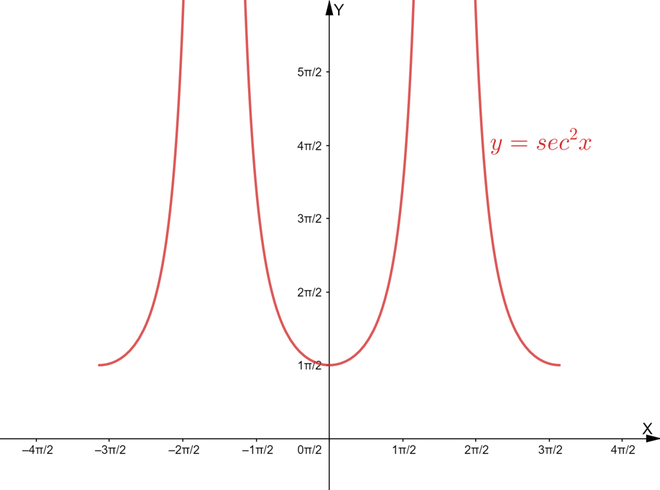Open in App
Not now

# Secant Square x Formula

• Last Updated : 31 May, 2022

Trigonometry is one of the most significant topics of mathematics, with numerous applications in a wide range of fields. The study of the connection between the sides and angles of a right-angle triangle is the main focus of trigonometry. As a result, using trigonometric formulae, functions, or trigonometric identities, it is possible to find the missing or unknown angles or sides of a right triangle. Angles in trigonometry can be measured in degrees or radians.

### Secant Trigonometric Ratio

The ratio of the lengths of any two sides of a right triangle is called a trigonometric ratio. In trigonometry, these ratios link the ratio of sides of a right triangle to the angle. The secant ratio is expressed as the ratio of the hypotenuse (longest side) to the side corresponding to a given angle in a right triangle. It is the reciprocal of the cosine ratio and is denoted by the abbreviation sec.If θ is the angle that lies between the base and hypotenuse of a right-angled triangle then,

sec θ = Hypotenuse/Base = 1/cos θ

Here, hypotenuse is the longest side of right triangle and base is the side adjacent to the angle.

### Secant Square x Formula

The secant square x ratio is denoted by the abbreviation sec2 x. It’s a trigonometric function that returns the square of the secant function value for an angle x. The period of the function sec x is 2π, but the period of sec2 x is π. Its formula is equivalent to the sum of unity and the tangent square function.Formula

sec2 x = 1 + tan2 x

where,

x is one of the angles of the right triangle,

tan x is the tangent ratio for angle x.

Derivation

The formula for secant square x is derived by using the identity of sum of squares of sine and cosine ratios.

We know, sin2 x + cos2 x = 1.

Dividing both sides by cos2 x, we get

(sin2 x/cos2 x) + (cos2 x/cos2 x) = 1/cos2 x

tan2 x + 1 = sec2 x

=> sec2 x = 1 + tan2 x

This derives the formula for secant square x ratio.

### Sample Problems

Problem 1. If tan x = 3/4, find the value of sec2 x using the formula.

Solution:

We have, tan x = 3/4.

Using the formula we get,

sec2 x = 1 + tan2 x

= 1 + (3/4)2

= 1 + 9/16

= 25/16

Problem 2. If tan x = 12/5, find the value of sec2 x using the formula.

Solution:

We have, tan x = 12/5.

Using the formula we get,

sec2 x = 1 + tan2 x

= 1 + (12/5)2

= 1 + 144/25

= 169/25

Problem 3. If sin x = 8/17, find the value of sec2 x using the formula.

Solution:

We have, sin x = 8/17.

Find the value of cos x using the formula sin2 x + cos2 x = 1.

cos x = √(1 – (64/289))

= √(225/289)

= 15/17

So, tan x = sin x/cos x = 8/15

Using the formula we get,

sec2 x = 1 + tan2 x

= 1 + (8/15)2

= 1 + 64/225

= 289/225

Problem 4. If cot x = 8/15, find the value of sec2 x using the formula.

Solution:

We have, cot x = 8/15.

So, tan x = 1/cot x = 15/8

Using the formula we get,

sec2 x = 1 + tan2 x

= 1 + (15/8)2

= 1 + 225/64

= 289/64

Problem 5. If cos x = 12/13, find the value of sec2 x using the formula.

Solution:

We have, cos x = 12/13.

Find the value of sin x using the formula sin2 x + cos2 x = 1.

sin x = √(1 – (144/169))

= √(25/169)

= 5/13

So, tan x = sin x/cos x = 5/12

Using the formula we get,

sec2 x = 1 + tan2 x

= 1 + (5/12)2

= 1 + 25/144

= 169/144

My Personal Notes arrow_drop_up